Search
 Submission Procedure share: |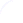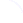available in:   PDF (115 kB) PS (25 kB)

get:
 Similar Docs BibTeX Write a comment
get:

DOI:   10.3217/jucs-001-03-0201

# Halting Probability Amplitude of Quantum Computers

K. Svozil
Institute for Theoretical Physics, Technische Universitaet Wien,
Wiedner Hauptstrasse 8-10/136, A-1040 Vienna, Austria
e-mail:svozil@tph.tuwien.ac.at

Abstract: The classical halting probabilityintroduced by Chaitin is generalized to quantum computations.

(The quantum omega was invented in a meeting of G. Chaitin, A. Zeilinger and the author (K. S.) in a Viennese coffee house (Cafe Braeunerhof) in January 1991. Thus, the group should be credited for the original invention, whereas any blame should remain with the author.)

# 1 Halting Probability Amplitude of Quantum Computers

Chaitin's,, is a magic number. It is a measure for arbitrary programs to take a finite number of execution steps and then halt. It contains the solution for all halting problems, and hence to questions codable into halting problems, such as Fermat's theorem. It contains the solution for the question of whether or not a particular exponential Diophantine equation has infinitely many or a finite number of solutions. And, sinceis provable 'algorithmically incompressible,' it is Martin-Loef/Chaitin/Solovay random. Therefore,is both: a mathematicians 'fair coin,' and a formalist's nightmare.

Here,is generalized to quantum computations.

Consider a (not necessarily universal) quantum computer C and its ith program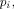which, at timecan be described by a quantum state , , , , , , , ,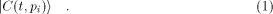A typical realisation of C would be by an array of generalized four-port beam splitters .

In what follows we shall assume that the program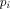is coded classically. That is, we choose a finite code alphabet A and denote by A* the set of all strings over A. Any programis coded as a classical sequence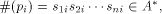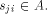(In what follows,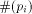will be abbreviated by.) We assume prefix coding , , , ; i.e., the domain of C is prefix-free such that no admissible program is the prefix of another admissible program. Furthermore, without loss of generality, we consider only empty input strings.

Page 201

In quantum information theory, the state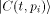of the computer C with programis representable by quantum bits (qbits). Every qbit is in a coherent superposition of the classical bit basis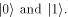Assume that the states (1) are orthogonal and normalized. The computer C evolves according to a unitary operator U such that (discrete time evolution)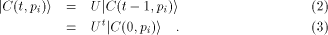More specifically, a quantum computer can be in a coherent superposition of the halting and the non-halting state ,We shall call this quantum bit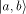the halting bit. The symbols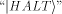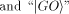represent orthonormal vectors  in the twodimensional complex Hilbert space spanned by them. Let the halting state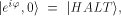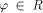be the physical realization that the computer has 'halted;' likewise let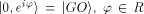be the physical realization that the computer has not `halted'. Note that, since quantum computations are governed by unitary evolution laws which are reversible, the halting state does not mean that the computer does not change as time evolves. It just means that it has set a signal --- the halting bit --- to indicated that it has finished its task. a and b are complex numbers which are a quantum mechanical measure of the probability amplitude that the computer is in the halting and the non-halting states, respectively. (The corresponding probabilities are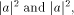respectively.) One important feature of the quantum information concept ist that it does not merely allow two-valued states but a coherent superposition thereof.

In the orthonormal halting basis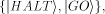the computer C with classical inputcan be represented byIn the spirit of quantum recursion theory , assume that initially, i.e., at time t=0, the computer is prepared such that its halting bit is in a coherent 50:50-superposition; i.e., in terms of the halting basis,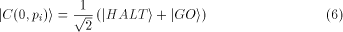for all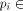A*. This corresponds to the fact that initially it is unknown whether or not the computer halts on. When during the time evolution the computer has completed its task, the halting bit value is switched to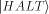by some internal operation, otherwise it remains in the coherent 50:50-superposition of equation (6). (Alternatively, the computer could be initially prepared in the non-halting state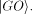After completion of the task, the halting bit is again switched to the halting state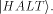In this case, the subtractions of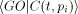in equations (7), (8) and (9) below would have to be eliminated.)

Page 202

In analogy to the fully classical case ,, the quantum halting amplitude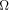can be defined as a weighted expectation over all computations of C with classical inputstands for the length of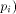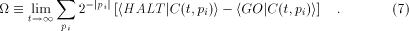Likewise, for particular output states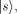For a set of output states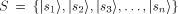which correspond to mutually orthogonal vectors in Hilbert space,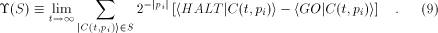For nontrivial choices of the quantum computer C, several remarks are in order. (In what follows, we mention only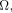but the comments apply toas well.)

First, note that if the program is also coded in qbits, the above sum becomes an integral over continuously many states per code symbol of the programs. In this case, the Kraft sum needs not converge.

Second, just as for the classical analogue it is possible to 'compute'as a limit from below by considering in the t'th computing step (time t) all programs of length t which have already halted. (This 'computation' suffers from a radius of convergence which decreases slower than any recursive function.)

Third, the quantumis complex.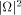can be interpreted as a measure for the halting probability of C; i.e., the probability that an arbitrary (prefix-free) program halts on C. Both, the relative phases of approximations of, as well as approximations toare measurable.

Finally, any measurements ofcauses irreversible state collapses. Sincemay not be in a pure state, the series in (7) and (8) will not be uniquely defined even for finite times. The nondeterministic character ofis not only based on classical recursion theoretic arguments  but also on the physical proposition that God plays the quantum dice.

 G. J. Chaitin, Information, Randomness and Incompleteness, Second edition (World Scientific, Singapore, 1987, 1990); Algorithmic Information Theory (Cambridge University Press, Cambridge, 1987); Information-Theoretic Incompleteness (World Scientific, Singapore, 1992).

 R. M. Solovay, unpublished manuscript.

Page 203

 C. Calude, Information and Randomness --- An Algorithmic Perspective (Springer, Berlin, 1994).

 D. Z. Albert, Phys. Lett. 94A, 249 (1983).

 P. Benioff, J. Stat. Phys. 29, 515 (1982); Phys. Rev. Lett. 48, 1581 (1982).

 D. Deutsch, Proc. R. Soc. Lond. A 400, 97 (1985).

 R. P. Feynman, Opt. News 11, 11 (1985).

 A. Peres, Phys. Rev. A32, 3266 (1985).

 P. Benioff, Annals New York Academy of Sciences 480, 475 (1986).

 N. Margolus, Annals New York Academy of Sciences 480, 487 (1986).

 D. Deutsch, Proc. R. Soc. Lond. A 425, 73 (1989).

 D. Deutsch and R. Jozsa, Proc. R. Soc. Lond. A 439, 553 (1992).

 A. Zeilinger, Am. J. Phys. 49, 882 (1981); M. Reck, A. Zeilinger, H. J. Bernstein and P. Bertani, Phys. Rev. Lett. 73, 58 (1994).

 R. W. Hamming, Coding and Information Theory, Second Edition (Prentice-Hall, Englewood Cliffs, New Jersey, 1980).

 K. Svozil, Randomness and Undecidability in Physics (World Scientific, Singapore, 1993).

 K. Svozil, On the computational power of physical systems, undecidability, the consistency of phenomena and the practical uses of paradoxa, in International Conference on Fundamental Problems in Quantum Theory, ed. by D. Greenberger (New York Academy of Sciences, in print); Quantum recursion theory, TU Vienna preprint.

 Let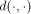be the inner product of the Hilbert space. Let further be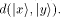. Orthonormality states that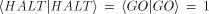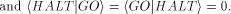R. J. Solomonoff, Information and Control 7, 1 (1964); IEEE Transactions on Information Theory IT-24, 422 (1978).

Page 204